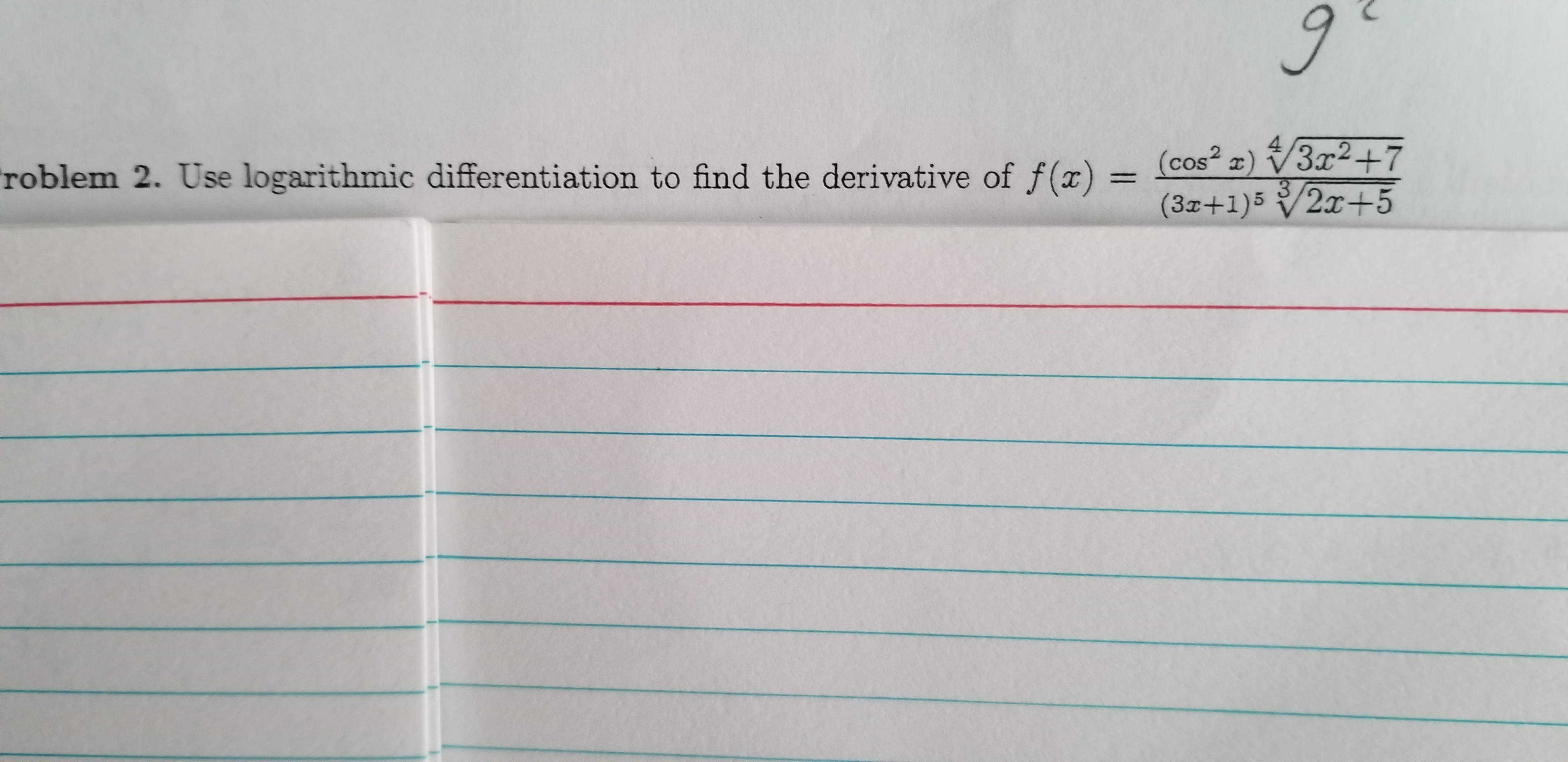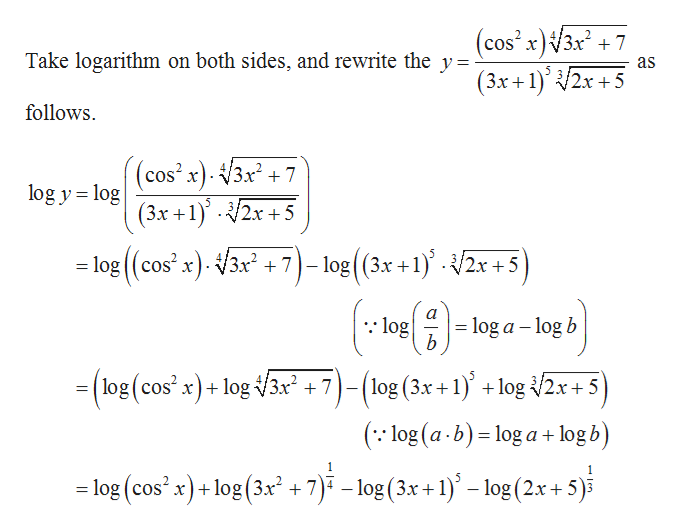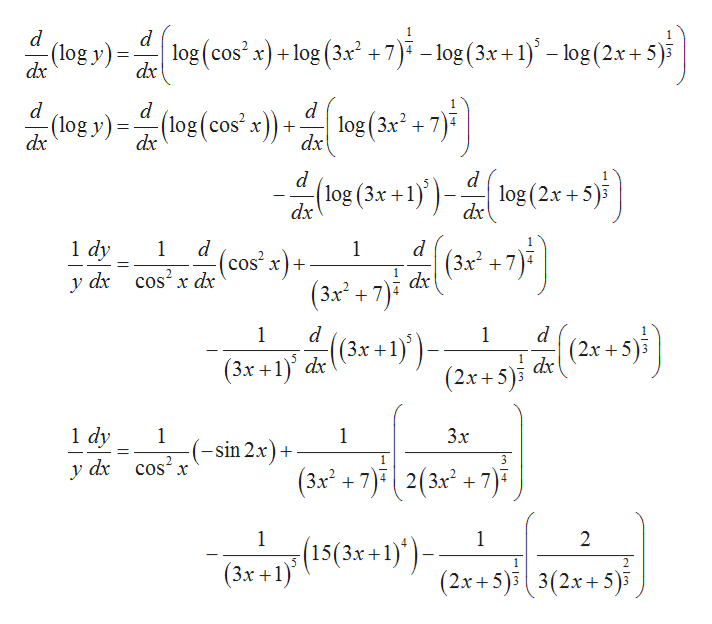# (cos² z) /3x²+73.(3x+1)5/2x+53x2+7roblem 2. Use logarithmic differentiation to find the derivative of f()

Question
1 viewshelp_outlineImage Transcriptionclose(cos² z) /3x²+7 3. (3x+1)5/2x+5 3x2+7 roblem 2. Use logarithmic differentiation to find the derivative of f() fullscreen
check_circle

Step 1help_outlineImage Transcriptionclose(cos x)V3x + 7 (3x+1)° 2x +5 Take logarithm on both sides, and rewrite the y= as follows. (cos' x). 3x² + 7 (3x +1)' - /2x + 5 log y = log = log ((cos² x). V3x + 7)– log(3.x +1)° · /2x +5 = log a – log b (log (cos² x)+ log /3x² + 7)- ) (log (3x+1) +log /2x+ 5 = (:: log (a -b) = log a + log b) 1. 7)" – log(3x +1)' – log (2x+ 5)i = log (cos? x)+ log(3x + %3D fullscreen
Step 2

Differentiate the above equation w...help_outlineImage Transcriptioncloselog (cos' x) + log (3x² +7) – log(3x + 1)° – log (2.x + 5)5 dx dx log y) = (log(cos" x)) + log(3x* + 7) log (3x? + 7) (log (3x +1)')– log (2x +5 dx 1 dy cos? x dx -(cos²x)+ | (3.x². dx y dx (3x + 7). |(2.x +5) (3x +1)° de (3r +1)')- (3x +1)' dx (2.x + 5)i de 1 dy Зх -(-sin 2x)+ сos? x у dх (3x² + 7)" 2(3x + 7 )7 5(15(3x+1)*)- (3x +1) - (2.x+5) 3(2x+5)5 fullscreen

### Want to see the full answer?

See Solution

#### Want to see this answer and more?

Solutions are written by subject experts who are available 24/7. Questions are typically answered within 1 hour.*

See Solution
*Response times may vary by subject and question.
Tagged in

### Calculus